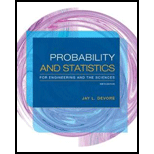# A transmitter is sending a message by using a binary code, namely, a sequence of 0’s and 1’s. Each transmitted bit (0 or 1) must pass through three relays to reach the receiver. At each relay, the probability is .20 that the bit sent will be different from the bit received (a reversal). Assume that the relays operate independently of one another. Transmitter → Relay 1 → Relay 2 → Relay 3 → Receiver a. If a 1 is sent from the transmitter, what is the probability that a 1 is sent by all three relays? b. If a 1 is sent from the transmitter, what is the probability that a 1 is received by the receiver? [ Hint: The eight experimental outcomes can be displayed on a tree diagram with three generations of branches, one generation for each relay.] c. Suppose 70% of all bits sent from the transmitter are 1s. If a 1 is received by the receiver, what is the probability that a 1 was sent?### Probability and Statistics for Eng...

9th Edition
Jay L. Devore
Publisher: Cengage Learning
ISBN: 9781305251809### Probability and Statistics for Eng...

9th Edition
Jay L. Devore
Publisher: Cengage Learning
ISBN: 9781305251809

#### Solutions

Chapter
Section
Chapter 2, Problem 94SE
Textbook Problem

## A transmitter is sending a message by using a binary code, namely, a sequence of 0’s and 1’s. Each transmitted bit (0 or 1) must pass through three relays to reach the receiver. At each relay, the probability is .20 that the bit sent will be different from the bit received (a reversal). Assume that the relays operate independently of one another.Transmitter → Relay 1 → Relay 2 → Relay 3 → Receiver a. If a 1 is sent from the transmitter, what is the probability that a 1 is sent by all three relays? b. If a 1 is sent from the transmitter, what is the probability that a 1 is received by the receiver? [Hint: The eight experimental outcomes can be displayed on a tree diagram with three generations of branches, one generation for each relay.] c. Suppose 70% of all bits sent from the transmitter are 1s. If a 1 is received by the receiver, what is the probability that a 1 was sent?

Expert Solution

a.

To determine

Find the probability that 1 is sent by all the three relays if 1 is sent from the transmitter.

### Explanation of Solution

Given info:

The message was sent by the transmitter using binary code in the form of 0’s and 1’s. Each transmitted binary code must cross three relays to reach the receiver. There is 20% chance that the probability of bit sent is different from the bit received. It should be assumed that the relays are independent of one another. The transmission of the message is explained as follows:

Calculation:

Define the event:

‘0’and‘1’ representsthe messages sent by the transmitter to the receiver.

Reversal bit denotes the event that the bit sent will be different from the bit received.

Original bit denotes the event that the bit sent will be same as the bit received.

The probabilities of the events are given below:

P(Reversal bit)=0.2, P(Orginal bit)=0.8.

Multiplication rule for two independent events:

If the events A and B are independent then,

P(AB)=P(A)×P(B).

Bayes’ rule:

If A1,A2,...,Ak are k mutually exclusive and exhaustive events, such that the sum of the probability values of the events is 1 and there is an observed event B, then,

P(Aj|B)=P(AjB)P(B)=P(Aj)P(B|Aj)P(A1)P(B|A1)+P(A2)P(B|A2)+...+P(Ak)P(B|Ak)

Here, j=1,...,k.

Law of total probability:

It should be assumed that the event A1, A2, A3,…,Ak be mutually exclusive and exhaustive events.

For any event B,

The probability of B is obtained as given below:

P(B)=P(B|A1)P(A1)+P(B|A2)P(A2)+...+P(B|Ak)P(Ak)

The probability of receiving two reversal bit and original bit is given below:

P([relay 1 sentreversal bit][relay 2 sentreversal bit][relay 3 sentorignial bit])=P(1011)=P(reversal bit)×P(reversal bit)×P(orignal bit)=(0

Expert Solution

b.

To determine

Obtain the probability for receiving 1 by the receiver given that 1 sent by the transmitter.

Expert Solution

c.

To determine

Obtain the probability that 1 was sent by the transmitter given that 1 was received by the receiver.

### Want to see the full answer?

Check out a sample textbook solution.See solution

### Want to see this answer and more?

Bartleby provides explanations to thousands of textbook problems written by our experts, many with advanced degrees!

See solution